### Jian Yang, Hong S. He, and Stephen R. Shifley. 2008. Spatial controls of occurrence and spread of wildfires in the Missouri Ozark Highlands. Ecological Applications 18:1212–1225.

Appendix A. Separate the contribution of vegetation type to fire occurrence from other factors in calculating fire initiation probability and fire ignition rate.

Statement of the problem

The landscape fire succession simulation model, LANDIS, simulates fire occurrence based on a statistical association of fire occurrence density (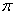) with a set of spatial covariates (Z) including road proximity, distance to nearest city, land ownership, topography, and vegetation type. Fire occurrence densityfor any location u on the landscape is specified through a log-linear Poisson process model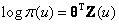(A.1)

Where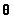is the vector of coefficients to be estimated for each of the corresponding covariates, and T is the matrix transpose operation. Among all of the above factors, only the change of vegetation type over time is explicitly simulated in LANDIS. Other factors are assumed to be constant over time in the model. Therefore, separating the contribution of vegetation to fire occurrence from the contribution of other factors can improve the model's computing performance in two aspects: (1) computational memory load can be greatly reduced when several maps of spatial covariates (e.g., slope, elevation, aspect, distance to nearest city) are replaced with only a map of fire ignition rate in the simulation to account for the contribution of all factors other than vegetation type; (2) computational cost can also be reduced when the calculation of fire occurrence density is simplified into the result of the two contributions.

Let the vector of coefficients () in Eq. A.1 to be a set of two components: a vector (P) of fire initiation probability and a vector (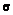) containing all other coefficients. When written in the matrix format, it is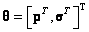. Furthermore, we write the list of spatial covariates (Z) into two parts: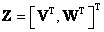, where V is the vector indicating which vegetation type the location u belongs to, and W is the vector containing all other spatial covariates. Then Eq. A.1 can be written as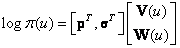(A.2)

Simplifying the matrix operation on the right hand side of the Eq. A.2, we get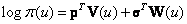(A.3)

Taking the exponential function transformation on both sides, we get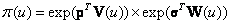(A.4)

Equation A.4 essentially indicates that fire occurrence density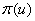is determined by two components (i.e., contribution from vegetation type and contribution from other factors). This is consistent with the hierarchical fire frequency model shown in our paper as fire occurrence density is a product of fire initiation probability p and ignition rate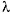. That is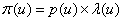(A.5)

Comparing A.4 and A.5, we decided to assume fire initiation probability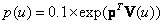(A.6)

and fire ignition rate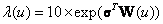(A.7)

Notice that we multiplied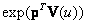by the coefficient 0.1 to normalize the value into the range of 0 and 1.

Estimation of fire initiation probability and fire ignition rate map

The coefficients estimated from the log-linear Poisson model and fire initiation probabilities for different vegetation types are shown in Table A1. The final model included polynomial transformed variables (e.g., D2) of some continuous covariates (e.g., road proximity, D). Fire initiation probabilities for vegetation types (Table A1) were calculated using Eq. A.4. Fire ignition rate was calculated using Eq. A.5. The derived map of ignition rate (Fig. A1) depicts the contribution of all other factors including road proximity, distance to nearest city, ownership, slope, and aspect. The estimated fire initiation probabilities with respect to different vegetation types and spatially explicit fire ignition rate map were used as parameters and input data in the LANDIS simulation.

TABLE A1. Estimated coefficients of the predictor variables used in the final log-linear Poisson model, and the corresponding fire initiation probabilities for the vegetation types.

 Variable Coefficient Fire initiation probability Vegetation type Grassland 0.591 0.181 Open woodland 0.789 0.220 Deciduous forest 0.857 0.236 Oak-pine mixed forest 0.870 0.239 Road proximity D:distance to nearest road (m) -1.6e-03 D2 3.2e-07 Ownership Private inholdings 2.6e+00 Public lands 3.2e+00 Slope S: Slope (degeree) 2.0e-02 S2 -6.6e-04 Aspect Xeric 1.4e-01 Flat 4.5e-01 Municipality proximity T:Distance to nearest towns (m) -6.4e-05 T2 -9.8e-09 T3 3.2e-13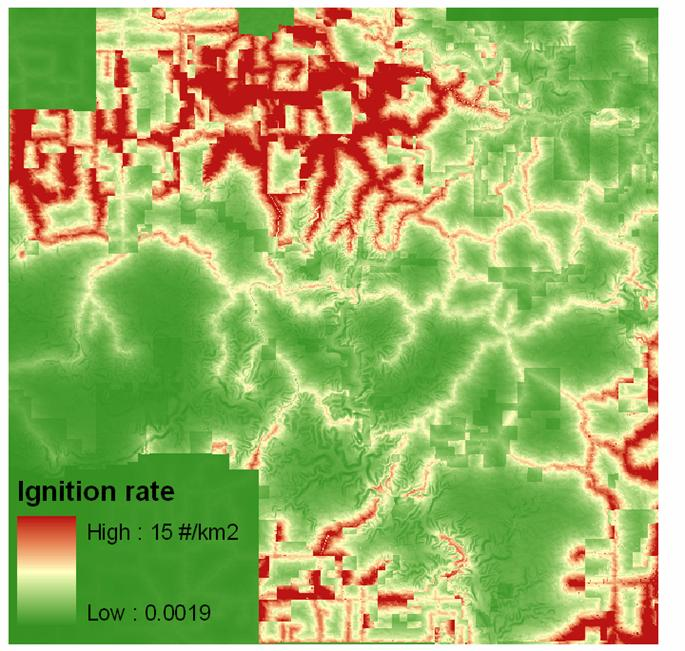FIG. A1. The map of estimated fire ignition rate, defined as the number of potential ignitions per square kilometer per decade.

[Back to A018-044]